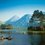# wierd proofs

$To\quad Prove\quad :\quad Any\quad power\quad of\quad 1\quad is\quad 1\\ we\quad all\quad are\quad aware\quad of\quad the\quad fact\quad that\\ { ({ a }^{ m }) }^{ n }\quad =\quad { ({ a }^{ n }) }^{ m }\\ Let\quad us\quad put\quad m\quad =\quad 0,\quad then\quad \\ { ({ a }^{ 0 }) }^{ n }\quad =\quad ({ something) }^{ 0 }\\ { 1 }^{ n }\quad =\quad 1\\ Hence\quad proved.$ Proof of the assumption I have used in the proof. ${ a }^{ 0 }\quad =\quad { a }^{ b-b }\\ { a }^{ b-b }\quad =\quad \frac { { a }^{ b } }{ { a }^{ b } } \quad =\quad \boxed { 1 }$ Credit to @ArchitBoobnaNote by Rajdeep Dhingra
6 years, 5 months ago

This discussion board is a place to discuss our Daily Challenges and the math and science related to those challenges. Explanations are more than just a solution — they should explain the steps and thinking strategies that you used to obtain the solution. Comments should further the discussion of math and science.

When posting on Brilliant:

• Use the emojis to react to an explanation, whether you're congratulating a job well done , or just really confused .
• Ask specific questions about the challenge or the steps in somebody's explanation. Well-posed questions can add a lot to the discussion, but posting "I don't understand!" doesn't help anyone.
• Try to contribute something new to the discussion, whether it is an extension, generalization or other idea related to the challenge.
• Stay on topic — we're all here to learn more about math and science, not to hear about your favorite get-rich-quick scheme or current world events.

MarkdownAppears as
*italics* or _italics_ italics
**bold** or __bold__ bold
- bulleted- list
• bulleted
• list
1. numbered2. list
1. numbered
2. list
Note: you must add a full line of space before and after lists for them to show up correctly
paragraph 1paragraph 2

paragraph 1

paragraph 2

[example link](https://brilliant.org)example link
> This is a quote
This is a quote
    # I indented these lines
# 4 spaces, and now they show
# up as a code block.

print "hello world"
# I indented these lines
# 4 spaces, and now they show
# up as a code block.

print "hello world"
MathAppears as
Remember to wrap math in $$ ... $$ or $ ... $ to ensure proper formatting.
2 \times 3 $2 \times 3$
2^{34} $2^{34}$
a_{i-1} $a_{i-1}$
\frac{2}{3} $\frac{2}{3}$
\sqrt{2} $\sqrt{2}$
\sum_{i=1}^3 $\sum_{i=1}^3$
\sin \theta $\sin \theta$
\boxed{123} $\boxed{123}$

## Comments

Sort by:

Top Newest

How will you prove that 1^n=1 without proving a^0=1

- 6 years, 5 months ago

Log in to reply

${ a }^{ 0 }\quad =\quad { a }^{ b-b }\\ { a }^{ b-b }\quad =\quad \frac { { a }^{ b } }{ { a }^{ b } } \quad =\quad \boxed { 1 }$

- 6 years, 5 months ago

Log in to reply

Your explanation for a^0 is correct,but you have to prove this in your note.

- 6 years, 5 months ago

Log in to reply

Rajdeep, this proof is created by me, so please mention it.

- 6 years, 5 months ago

Log in to reply

Of course, we're making the assumption that $0^{0}$ is 1, correct?

- 6 years, 5 months ago

Log in to reply

No we are not... we can consider a is not equal to 0... instead of "a" we could have used any constant

- 6 years, 5 months ago

Log in to reply

Alright, just making sure...

- 6 years, 5 months ago

Log in to reply

@Archit Boobna Whats ur score in NTSE Delhi held on 18 Dec. Btw what is score highest in FSD?

- 4 years, 5 months ago

Log in to reply

No we are not Please tell in which step am i using it

- 6 years, 5 months ago

Log in to reply

×

Problem Loading...

Note Loading...

Set Loading...